# Theory

## Size and Distance in the Sky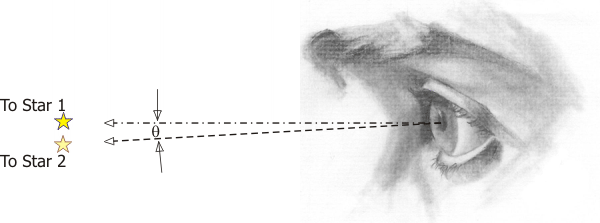Everything in the night sky is so far away that it’s not the actual distances between things that we see, it’s the differences in the angle from our vantage point.

For that reason we measure distances and sizes in the scope image in terms of angles – degrees and fractions of degrees. The actual system is not degrees and decimal degrees, but instead it’s degrees, arc-minutes, and arc-seconds, which works just like hours, minutes & seconds. So there are

• 60 arc-minutes to a degree (just like 60 minutes to an hour).
• 60 arc-seconds to an arc-minute (just like 60 seconds to a minute).

Then if I am looking at two stars, the distance between them is expressed as an angle, in degrees, or in arc-minutes, or in arc-seconds. In the equations, we use θ (the Greek letter "theta") to represent angles.

When the stars are very close, it is common for us to measure their separation in terms of just a few arc-seconds, which is one 3600th of a degree. That works out to about one-fourth of one-thousanth of a degree!

## First an Intuitive Look at Magnification

In spite of all the diagrams and equations, what a telescope does to magnify the images is not magic or even complicated. It is actually quite simple and intuitive.

The objective lens brings the image to a focus at its focal length. When viewed from the position of the objective lens, this image of the sky is the same size as the eye's view of the sky -- that is, distance angles between stars are exactly the same as you see them when you look up.

Then the eyepiece, with a much shorter focal length, lets you get very close to that image to look at it, and -- surprise -- when you get closer, the image is bigger. If the eyepiece lets you get twice as close, the image is twice as big. If the eyepiece lets you get 50 times closer, then the image is 50 times as big. Simple.

## Effect of the Eyepiece

In theory, we could change the magnification either by changing the focal length of the objective lens or by changing that of the eyepiece. In reality, the objective is permanently mounted in the scope and we're not about to change it. To change magnification, we will change the eyepiece.

Notice, though, that based on the discussion above, different focal length eyepieces really are just moving you closer to, or further from, the image plane of the objective. When you get closer, the image is bigger (higher magnification) and when you get further, the image is smaller (lower magnification). This is a very effective (and very accurate) way of thinking about how the scope works.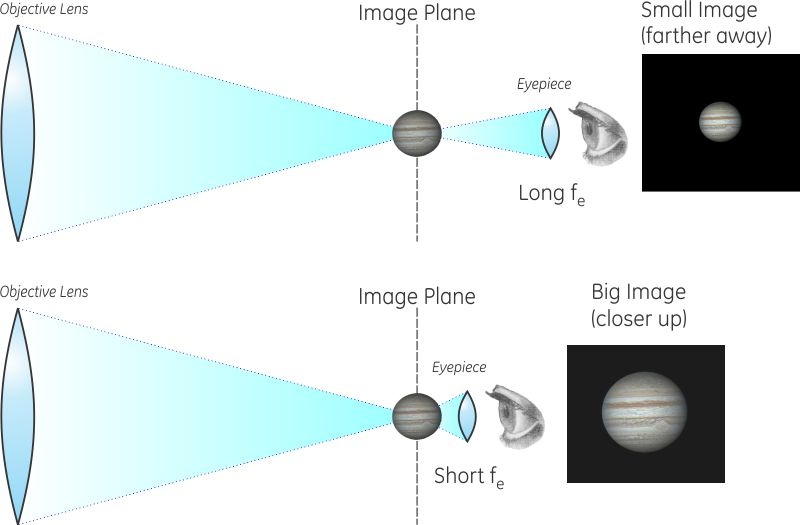So I control how big the image looks simply by getting closer to or further from the image. Now let's see how the mathematics say exactly the same thing.

## Magnification Equation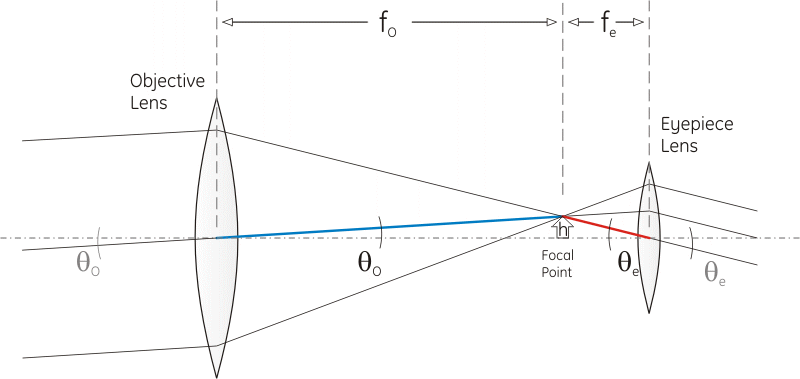As the image gets magnified for the observer, the position of each feature in the image moves to a larger and larger angle off the centerline (i.e. the line looking straight ahead). Hence the magnification can be figured as the ratio of the angle seen at the eyepiece to the angle seen by the objective lens. For our analysis let's define some terms:

Angle seen at objective = θO
Angle seen at eyepiece = θe

We will also define the focal length of each lens, that is, the distance from the lens where it focuses light to a point.

Focal length of objective = fO
Focal length of eyepiece = fe

We can use the diagram above to find the magnification for this telescope. Light rays from a distant point arrive at the objective in parallel. The ray that passes through the center of the lens is important for the analysis, because that ray (unlike the others) is not bent and forms a straight line through the lens.

Then the angle of this incoming ray from the centerline, θO, is the same at the front and the back of the lens. Follow this line (the blue line) from the center of the objective to the focal point. The focal point, by definition, sits at distance fO from the objective, and we will postulate that this particular point sits at distance h above the centerline at the focal plane.

We can then find θO, in radians, as approximately equal to h/fO.

Now follow the red line from the focal plane to the center of the eyepiece and you can see that, likewise, the angle at the eyepiece, θe can be found as h/fe.

Then the telescope's magnification will be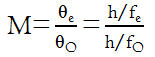and after we cancel the h's in top & bottom and rearrange we get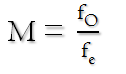See? It's how far the objective is from the image compared to how close the eyepiece can get to it. You get closer and the image gets bigger.

The Minimum Magnification page explains how you can determine magnification from the exit pupil, and the Surface Brightness page explains why you would care about something like that.

## Field of View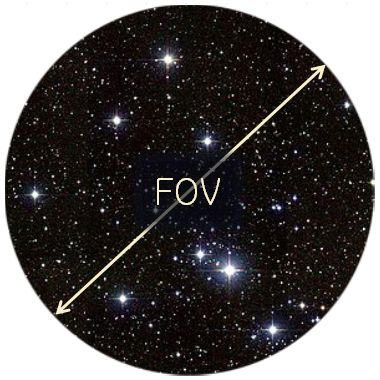Notice above in the "small image" I can see more of the sky around Jupiter, and in the "big image", I can see less of the sky around it. The span — distance across the image from one side to the other — is bigger at low magnification and smaller at high magnification. We refer to this span as the "field of view". Let's figure out how big that actually is.

If you hold up your eyepiece by itself and look through it, assuming you don't go cross-eyed trying, you will see that there is an upside-down image. The width of that image, measured as an angle, is the field of view of the eyepiece. Typically, an eyepiece has a field of view of 50-60°, although there are wide-field eyepieces that go up to as much as 82° (and higher -- I just saw one that has a field of 100°!).

When you expand the image by magnification, you can no longer see the whole field that you could see before magnification. So for example if you magnify the image by a factor of ten, you now can only see one-tenth of the field you had without magnification.

So to find what the field of view will be in your telescope, first find the field of view of the eyepiece from its specifications, then divide by the magnification of your scope (with that eyepiece). Expressed as an equation this comes out to be## Image Resolution

What is the tiniest detail I can see at this magnification? We usually describe this in terms of how close two stars can be together and still tell them apart as two stars. We're also usually talking pretty darn close, so it is measured in arcseconds.

In the section on Maximum Magnification it's shown that a person with 20/20 vision can tell apart two stars that are as close as 120 arcseconds. So if you want to be able to see two stars that are much closer than that, the telescope will need to magnify them up to be 120 arcseconds apart. How close can they be if my magnification is M? What resolution, when magnified by M, will be 120 arcseconds?

M*Resolution = 120, therefore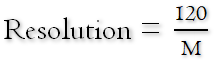# Practice

## Calculating the Scope's Magnification

Once you understand the principle, the calculation is pretty easy. Don't underestimate the importance of being able to find this number, though, it is fundamental to the determination of most other performance parameters of the telescope.

So let's try a couple of quick examples.

My first telescope was a Meade 6600 -- they don't make it any more -- it's a 6-inch f/5 Newtonian scope. It came with a 25mm eyepiece. So... what was the magnification I was getting with this scope?

Incidentally, notice how I characterize the scope and the eyepiece... the scope by its diameter and f-ratio, the eyepiece by its focal length. This is common parlance. It's also common to call out the scope diameter in inches, but for our purposes we will need to convert to mm. So the value we want for DO is 6 × 25.4 = 152.4mm.

Wait a minute... the magnification is the focal length of the objective divided by the focal length of the eyepiece... so -- don't I need to tell you the focal length of the objective? Ah, but I have. Since the f-ratio is the focal length of the objective divided by the diameter of the objective, fR = fO/DO, then the focal length of the objective is found from

fO = DO×fR = 152.4 × 5 = 762 mm.

Then the magnification is fO/fe = 762/25 = 30.48, which we would just call 30.

The eyepiece has a field of view of 52°, so the field of view for the telescope at this magnification will be 52 ÷ 30 = 1.7°.

Then the image resolution at this magnification = 120/30 = 4 arcseconds. I would just be able to split a double star that is 4 arcseconds apart, like gamma Leonis.

## Dependence on the Eyepiece

Since I got this scope, I've gotten a number of eyepieces so I can work at different magnifications. I have a 15 mm, a 9 mm, and a 4 mm eyepiece, in addition to the 25 mm. Let's see what these do for me in magnification and field of view, given the eyepieces each have a field of view of 52°:

Eyepiece Arithmetic Magnification Field of View Resolution
25 mm 762/25 = 30 1.7° 4 arcsec
15 mm 762/15 = 50 1.0° 2.4 arcsec
9 mm 762/9 = 85 0.6° 1.4 arcsec
4 mm 762/4 = 190 0.3° 0.6 arcsec

Later on, where we talk about minimum and maximum magnification, we examine this set of eyepieces and how well matched it is to this scope.

## Dependence on the Scope

I also have a 90mm f/13.9 Meade ETX, which came with a 26mm eyepiece. What is the magnification on this much smaller scope?

Same arithmetic as above:

fO = DO×fR = 90 × 13.9 = 1250 mm.

Then the magnification is fO/fe = 1250/26 = 48.

So the larger diameter scope, with a low f-ratio, has a much lower magnification than the small diameter scope, with a much bigger f-ratio. In general this will be the case -- high f-ratio tends to mean high magnification, and low f-ratio tends to mean low magnification. So why in the world would someone want a scope with a low f-ratio?

To understand the answer to that question, you need to dive into the discussion on Surface Brightness.

## Questions

Your questions and comments regarding this page are welcome. You can e-mail Randy Culp for inquiries, suggestions, new ideas or just to chat.
Updated 11 May 2019